Rudiments of Hierarchical Clustering: Ward’s Method and Divisive Clustering

# Rudiments of Hierarchical Clustering: Ward’s Method and Divisive Clustering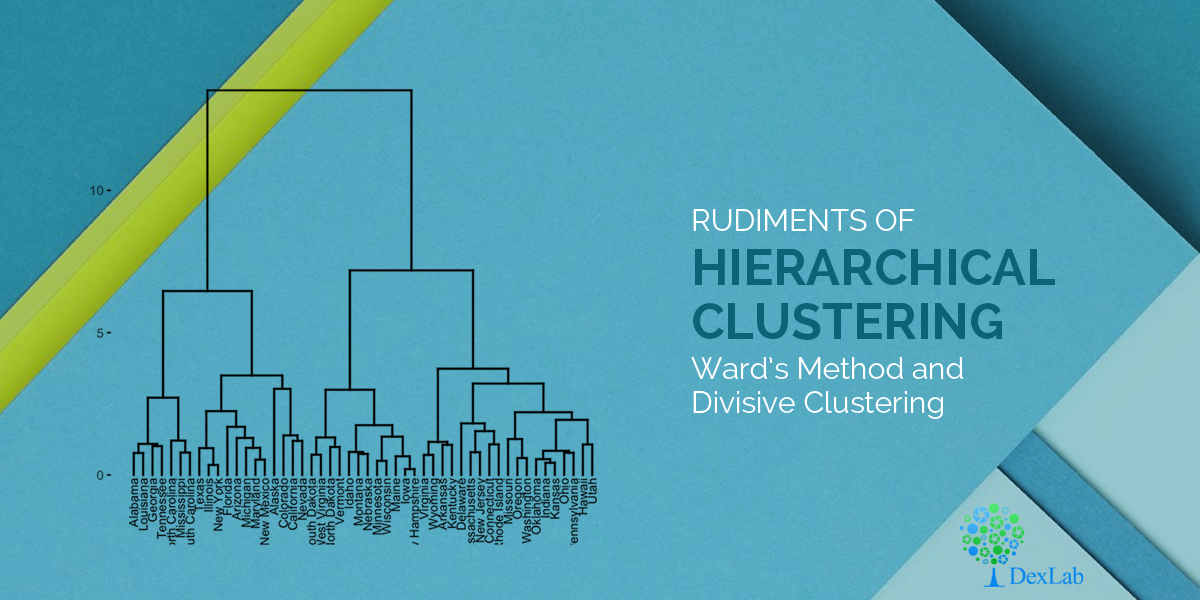Clustering, a process used for organizing objects into groups called clusters, has wide ranging applications in day to day life, including fields like marketing, city-planning and scientific research.

Hierarchical clustering, one the most common methods of clustering, builds a hierarchy of clusters either by a ‘’bottom up’’ approach (Agglomerative clustering) or by a ‘’top down’’ approach (Divisive clustering). In the previous blogs, we have discussed the various distance measures and how to perform Agglomerative clustering using linkage types. Today, we will explain the Ward’s method and then move on to Divisive clustering.

#### Ward’s method:

This is a special type of agglomerative hierarchical clustering technique that was introduced by Ward in 1963. Unlike linkage method, Ward’s method doesn’t define distance between clusters and is used to generate clusters that have minimum within-cluster variance. Instead of using distance metrics it approaches clustering as an analysis of variance problem. The method is based on the error sum of squares (ESS) defined for jth cluster as the sum of the squared Euclidean distances from points to the cluster mean.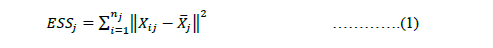Where Xij is the ith observation in the jth cluster. The error sum of squares for all clusters is the sum of the ESSj values from all clusters, that is,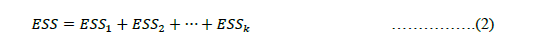Where k is the number of clusters.

The algorithm starts with each observation forming its own one-element cluster for a total of n clusters, where n is the number of observations. The mean of each of these on-element clusters is equal to that one observation. In the first stage of the algorithm, two elements are merged into one cluster in a way that ESS (error sum of squares) increases by the smallest amount possible. One way of achieving this is merging the two nearest observations in the dataset.

Up to this point, the Ward algorithm gives the same result as any of the three linkage methods discussed in the previous blog. However, as each stage progresses we see that the merging results in the smallest increase in ESS.

This minimizes the distance between the observations and the centers of the clusters. The process is carried on until all the observations are in a single cluster.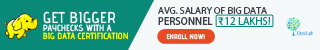#### Divisive clustering:

Divisive clustering is a ‘’top down’’ approach in hierarchical clustering where all observations start in one cluster and splits are performed recursively as one moves down the hierarchy. Let’s consider an example to understand the procedure.

Consider the distance matrix given below. First of all, the Minimum Spanning Tree (MST) needs to be calculated for this matrix.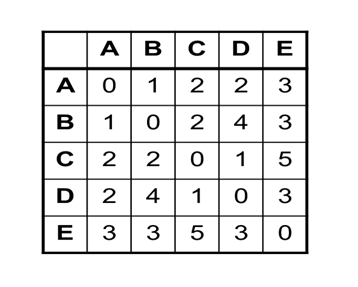The MST Graph obtained is shown below.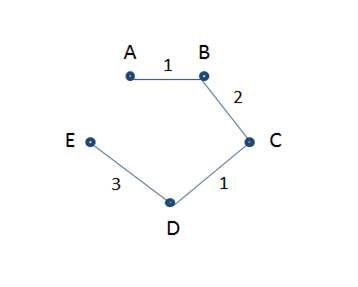The subsequent steps for performing divisive clustering are given below:

Cut edges from MST graph from largest to smallest repeatedly.

Step 1: All the items are in one cluster- {A, B, C, D, E}

Step 2: Largest edge is between D and E, so we cut it in 2 clusters- {E}, {A., B, C, D}

Step 3: Next, we remove the edge between B and C, which results in- {E}, {A, B} {C, D}

Step 4: Finally, we remove the edges between A and B (and between C and D), which results in- {E}, {A}, {B}, {C} and {D}

Hierarchical clustering is easy to implement and outputs a hierarchy, which is structured and informative. One can easily figure out the number of clusters by looking at the dendogram.

However, there are some disadvantages of hierarchical clustering. For example, it is not possible to undo the previous step or move around the observations once they have been assigned to a cluster. It is a time-consuming process, hence not suitable for large datasets. Moreover, this method of clustering is very sensitive to outlietrs and the ordering of data effects the final results.

In the following blog, we shall explain how to implement hierarchical clustering in R programming with examples. So, stay tuned and follow DexLab Analytics – a premium Big Data Hadoop training institute in Gurgaon. To aid your big data dreams, we are offering flat 10% discount on our big data Hadoop courses. Enroll now!

#### Check back for our previous blogs on clustering:

Hierarchical Clustering: Foundational Concepts and Example of Agglomerative Clustering